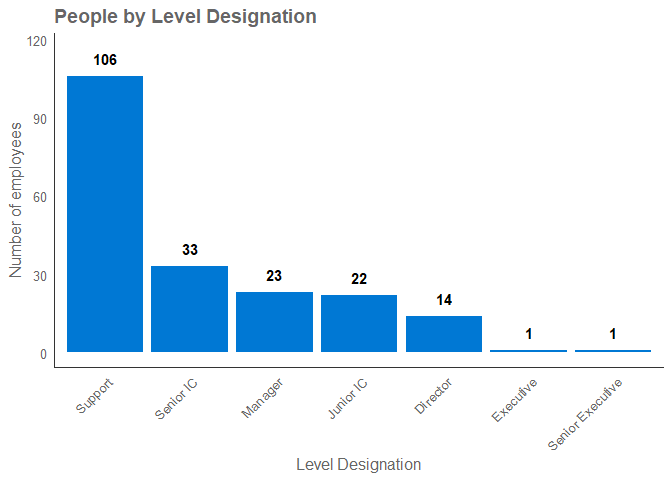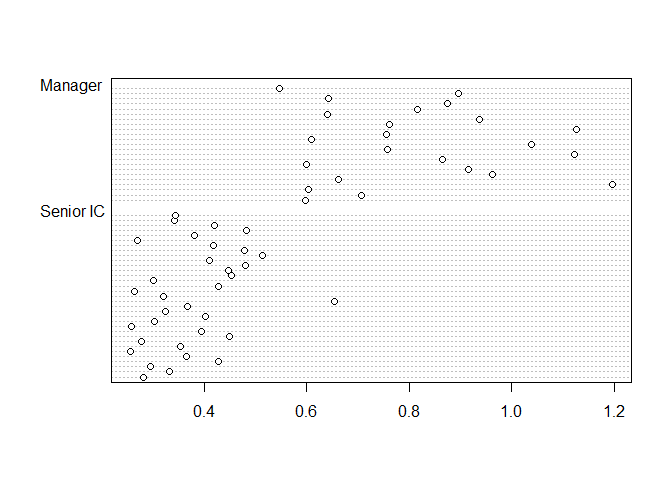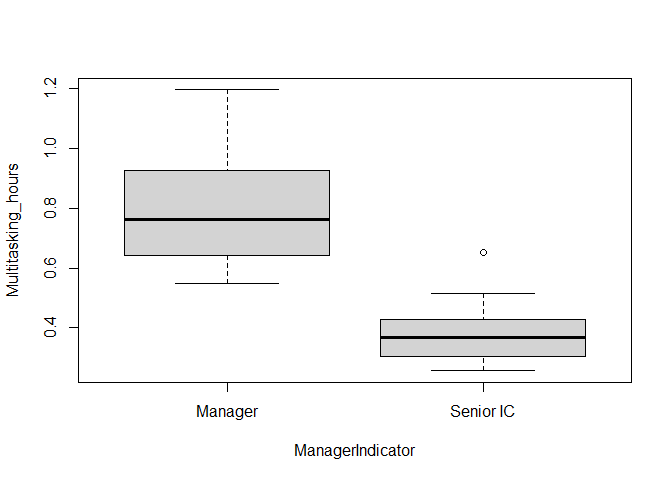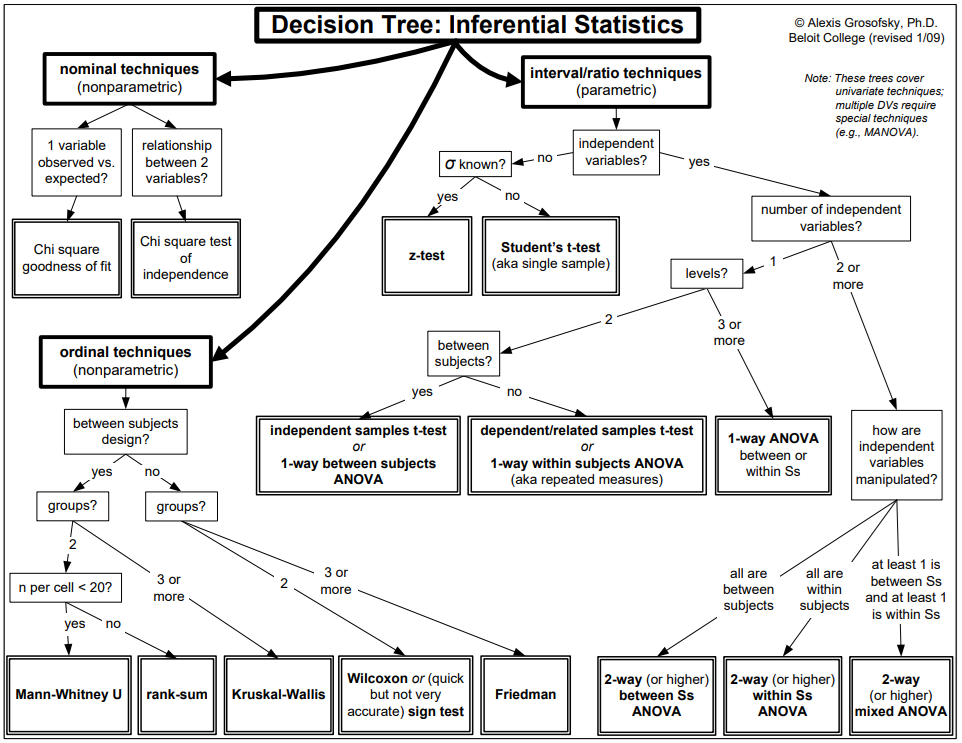# Musings on R

A blog on all things R and Data Science by Martin Chan

# Common Statistical Tests in R - Part I

Written on October 13, 2022

# Introduction

This post will focus on common statistical tests in R to understand and validate the relationship between two variables.

There must be tons of similar tutorials around, you may be thinking. So why?

The primary (and selfish) goal of the post is to create a guide that is practical enough for myself to refer to from time to time. This post is edited from my own notes from learning statistics and R, and have been applied to a data example/scenario that I am familiar with. This means that the examples should be easily generalisable and mostly consistent with my usual coding approach (mostly ‘tidy’ and using pipes). Along the way, this will hopefully benefit others who are learning statistics and R too.image from Giphy

To illustrate the R code, I will be using a sample dataset pq_data from the package vivainsights, which is a cross-sectional time-series dataset measuring the collaboration behaviour of simulated employees in an organization. Each row represents an employee on a certain week, with columns measuring behaviours such as total weekly time spent in email, meetings, chats, and so on. The vivainsights package itself provides visualisation and analysis functions tailored for these datasets which are available from Microsoft Viva Insights.

A note about the structure of this post: in the real world, one should as a best practice visually check the data distribution and run tests for assumptions like normality prior to performing any tests. For the sake of narrative and covering all the scenarios, this practice isn’t really observed in this post. Hence, please be forgiving as you see us run ‘head first’ into a test without examining the data - and avoid this in real life!

# Set-up: packages and data

The package vivainsights is available on CRAN, so you can install this with install.packages("vivainsights").

You can load the dataset in R by calling pq_data after loading the vivainsights package. Here is a preview of the first ten columns of the dataset using dplyr::glimpse():

library(vivainsights)

glimpse(pq_data[, 1:10])
## Rows: 5,593
## Columns: 10
## $PersonId <chr> "2b625906-1f36-3273-8d0d-13e714c5f6~ ##$ MetricDate                         <date> 2021-12-26, 2021-12-26, 2021-12-26~
## $After_hours_call_hours <dbl> 0, 0, 0, 0, 0, 0, 0, 0, 0, 0, 0, 0,~ ##$ After_hours_chat_hours             <dbl> 0, 0, 0, 0, 0, 0, 0, 0, 0, 0, 0, 0,~
## $After_hours_collaboration_hours <dbl> 7.6624994, 2.4908612, 0.1625000, 1.~ ##$ After_hours_email_hours            <dbl> 0.2600000, 0.5883611, 0.1625000, 0.~
## $After_hours_meeting_hours <dbl> 7.50, 2.00, 0.00, 1.25, 19.00, 0.25~ ##$ After_hours_scheduled_call_hours   <dbl> 0, 0, 0, 0, 0, 0, 0, 0, 0, 0, 0, 0,~
## $After_hours_unscheduled_call_hours <dbl> 0, 0, 0, 0, 0, 0, 0, 0, 0, 0, 0, 0,~ ##$ Call_hours                         <dbl> 0, 0, 0, 0, 0, 0, 0, 0, 0, 0, 0, 0,~

This tutorial also uses functions from tidyverse, so ensure that you run library(tidyverse) to reproduce the example outputs.

# Framing the problem

One of the most fundamental tasks in statistics and data science is to understand the relation between two variables. Sometimes the motivation is understand whether the relationship is causal, i.e. whether one causes another. This is not always the case, as for instance, one may simply wish to test for multicollinearity when selecting predictors for a model.1

Our dataset pq_data represents the simulated collaboration data of a company, and each row represents an employee’s week. There are two metrics of interest:

• Multitasking_hours measures the total number of hours the person spent sending emails or instant messages during a meeting or a Teams call.
• After_hours_collaboration_hours measures the number of hours a person has spent in collaboration (meetings, emails, IMs, and calls) outside of working hours.2

Imagine then we have two questions to address:

1. Do managers multi-task more than senior individual contributors (IC)?

2. The HR leadership suspects that meeting multitasking behaviour could be correlated with after-hours working, as the former represents wasted time and productivity during meetings. What can we do to understand the relationship between the two?

In this post, we will tackle the first question, and focus primarily on comparison tests and their non-parametric equivalents in R. In subsequent posts I would also like to cover other relevant tools/concepts such as correlation tests, regression tests, effect size, and statistical power.

It is worth noting that the first question postulates a relation between a categorical variable (manager/ senior IC) and a continuous variable (multitasking hours), whereas the second question a relation between two continuous variables (multitasking hours, afterhours collaboration). The types of the variables in question help determine which tests are appropriate.

The categorical variable that provides us information on whether an employee is a manager or a senior IC in pq_data is stored in LevelDesignation. We can use vivainsights::hrvar_count() to explore this variable:

hrvar_count(pq_data, hrvar = "LevelDesignation")# 1. Comparison tests: the t-test

Two common comparison tests would be the t-test and Analysis of Variance (ANOVA). The oft-cited practical difference between the two is that you would use the t-test for comparing means between two groups, and ANOVA for more than two groups. There is a bit more nuance than that, but we will start with the t-test.

A t-test can be paired or unpaired, where the former is used for comparing the means of two groups in the same population, and the latter for independent samples from two populations or groups. Since managers and senior ICs are two different populations, an unpaired (two-sample) t-test is therefore appropriate for the scenario in question two.

Before we jump into the test, we’ll need to prepare the data. Since we are interested in the difference between managers and senior ICs, we will first need to create a factor variable from the data that has only two levels. In the below code, we will first filter out any values of LevelDesignation that are not "Manager" and "Senior IC", and create a new factor column as ManagerIndicator:

pq_data_grouped <-
pq_data %>%
filter(LevelDesignation %in% c("Manager", "Senior IC")) %>%
mutate(
ManagerIndicator =
factor(LevelDesignation,
levels = c("Manager", "Senior IC"))
)

Recall also that our dataset pq_data is a cross-sectional time-series dataset, which means that for every individual identified by PersonId, there will be multiple rows representing a snapshot of a different week. In other words, a unique identifier would be something like a PersonWeekId. To simplify the dataset so that we are looking at person averages, we can group the dataset by PersonId and calculate the mean of Multitasking_hours for each person. After this manipulation, Multitasking_hours would represent the mean multitasking hours per person, as opposed to per person per week. Let us do this by building on the pipe-chain:

pq_data_grouped <-
pq_data %>%
filter(LevelDesignation %in% c("Manager", "Senior IC")) %>%
mutate(
ManagerIndicator =
factor(LevelDesignation,
levels = c("Manager", "Senior IC"))
) %>%
group_by(PersonId, ManagerIndicator) %>%

glimpse(pq_data_grouped)
## Rows: 56
## Columns: 3
## $PersonId <chr> "00f6d464-ba1f-31ee-b51e-ab6e8ec4fb79", "023ddb61-1~ ##$ ManagerIndicator   <fct> Senior IC, Manager, Senior IC, Senior IC, Manager, ~
groups = pq_data_grouped$ManagerIndicator )boxplot( Multitasking_hours ~ ManagerIndicator, data = pq_data_grouped )# 2. Non-parametric tests ## 2.1 Wilcoxon Rank-Sum Test Previously, we could not safely rely on the unpaired two-sample t-test because we are not fully confident that the data satisfies the normality condition. As an alternative, we can use the Wilcoxon Rank-Sum test (aka Mann Whitney U Test). The Wilcoxon test is described as a non-parametric test, which in statistics typically means that there is no specification on a distribution, or the parameters of a distribution. In this case, the Wilcoxon test does not assume a normal distribution. Another difference between the Wilcoxon Rank-Sum test and the unpaired t-test is that the former tests whether two populations have the same shape via comparing medians, whereas the latter parametric test compares means between two independent groups. This is run using wilcox.test() wilcox.test( Multitasking_hours ~ ManagerIndicator, data = pq_data_grouped, paired = FALSE ) ## ## Wilcoxon rank sum exact test ## ## data: Multitasking_hours by ManagerIndicator ## W = 752, p-value = 2.842e-14 ## alternative hypothesis: true location shift is not equal to 0 The p-value of the test is less than the significance level (alpha = 0.05), which allows us to conclude that Managers’ median multitasking hours is significantly different from the ICs’. Note that the Wilcoxon Rank-Sum test is different from the similarly named Wilcoxon Signed-Rank test, which is the equivalent alternative for the paired t-test. To perform the Wilcoxon Signed-Rank test instead, you can simply specify the argument to be paired = TRUE. Similar to the decision of whether to use the paired or the unpaired t-test, you should ensure that the one-sample condition applies if you use the Wilcoxon Signed-Rank test. ## 2.2 Kruskal-Wallis test So far, we have only been looking at tests which compare exactly two populations. If we are looking for a test that works with comparisons across three or more populations, we can consider the Kruskal-Wallis test. Let us create a new data frame that is grouped at the PersonId level, but filtering out fewer values in LevelDesignation: pq_data_grouped_2 <- pq_data %>% filter(LevelDesignation %in% c( "Support", "Senior IC", "Junior IC", "Manager", "Director" )) %>% mutate(ManagerIndicator = factor(LevelDesignation)) %>% group_by(PersonId, ManagerIndicator) %>% summarise(Multitasking_hours = mean(Multitasking_hours), .groups = "drop") glimpse(pq_data_grouped_2) ## Rows: 198 ## Columns: 3 ##$ PersonId           <chr> "0049ef24-ec83-356d-89f7-46b67364e677", "00f6d464-b~
## $ManagerIndicator <fct> Support, Senior IC, Manager, Support, Support, Supp~ ##$ Multitasking_hours <dbl> 0.3812649, 0.2813373, 0.5980080, 0.2918829, 0.42288~

We can then run the Kruskal-Wallis test:

kruskal.test(
data = pq_data_grouped_2
)
##
##  Kruskal-Wallis rank sum test
##
## Kruskal-Wallis chi-squared = 91.061, df = 4, p-value < 2.2e-16

Based on the Kruskal-Wallis test, we reject the null hypothesis and we conclude that at least one value in LevelDesignation is different in terms of their weekly hours spent multitasking. The most obvious downside to this method is that it does not tell us which groups are different from which, so this may need to be followed up with multiple pairwise-comparison tests (also known as post-hoc tests).

# 3. Comparison tests: ANOVA

## 3.1 ANOVA

What if we want to run the t-test across more than two groups?

Analysis of Variance (ANOVA) is an alternative method that generalises the t-test beyond two groups, so it is used to compare three or more groups.

There are several versions of ANOVA. The simple version is the one-way ANOVA, but there is also two-way ANOVA which is used to estimate how the mean of a quantitative variable changes according to the levels of two categorical variables (e.g. rain/no-rain and weekend/weekday with respect to ice cream sales). In this example we will focus on one-way ANOVA.

There are three assumptions in ANOVA, and this may look familiar:

• The data are independent.
• The responses for each factor level have a normal population distribution.
• These distributions have the same variance.

These assumptions are the same as those required for the classic t-test above, and it is recommended that you check for variance and normality prior to ANOVA.

ANOVA calculates the ratio of the between-group variance and the within-group variance (quantified using sum of squares), and then compares this with a threshold from the Fisher distribution (typically based on a significance level). The key function is aov():

res_aov <-
aov(
data = pq_data_grouped_2
)

summary(res_aov)
##                   Df Sum Sq Mean Sq F value Pr(>F)
## ManagerIndicator   4  40.55   10.14   504.6 <2e-16 ***
## Residuals        193   3.88    0.02
## ---
## Signif. codes:  0 '***' 0.001 '**' 0.01 '*' 0.05 '.' 0.1 ' ' 1

The interpretation is as follows:5

• Df: degrees of freedom for…

• the outcome variable, i.e. the number of levels in the variable minus 1
• the residuals, i.e. the total number of observations minus one and minus the number of levels in the outcome variables
• Sum Sq: sum of squares, i.e. the total variation between the group means and the overall mean

• Mean Sq: mean of the sum of squares, calculated by dividing the sum of squares by the degrees of freedom for each parameter

• F value: test statistic from the F test. This is the mean square of each independent variable divided by the mean square of the residuals. The larger the F value, the more likely it is that the variation caused by the outcome variable is real and not due to chance.

• Pr(>F): p-value of the F-statistic. This shows how likely it is that the F-value calculated from the test would have occurred if the null hypothesis of no difference among group means were true.

Given that the p-value is smaller than 0.05, we reject the null hypothesis, so we reject the hypothesis that all means are equal. Therefore, we can conclude that at least one value in LevelDesignation is different in terms of their weekly hours spent multitasking.

Antoine Soetewey’s blog recommends the use of the report package, which can help you make sense of the results more easily:

library(report)

report(res_aov)
## The ANOVA (formula: Multitasking_hours ~ ManagerIndicator) suggests that:
##
##   - The main effect of ManagerIndicator is statistically significant and large
## (F(4, 193) = 504.61, p < .001; Eta2 = 0.91, 95% CI [0.90, 1.00])
##
## Effect sizes were labelled following Field's (2013) recommendations.

The same drawback that applies to the Kruskall-Wallis test also applies to ANOVA, in that doesn’t actually tell you which exact group is different from which; it only tells you whether any group differs significantly from the group mean. This ANOVA test is hence sometimes also referred to as an ‘omnibus’ test.

## 3.2 Next steps after ANOVA

A pairwise t-test (note: pairwise, not paired!) is likely required to provide more information, and it is recommended that you review the p-value adjustment methods when doing so.6 Type I errors are more likely when running t-tests pairwise across many variables, and therefore correction is necessary. Here is an example of how you might run a pairwise t-test:

pairwise.t.test(
x = pq_data_grouped_2$Multitasking_hours, g = pq_data_grouped_2$ManagerIndicator,
paired = FALSE,
)
##
##  Pairwise comparisons using t tests with pooled SD
##
## data:  pq_data_grouped_2$Multitasking_hours and pq_data_grouped_2$ManagerIndicator
##
##           Director Junior IC Manager Senior IC
## Junior IC <2e-16   -         -       -
## Manager   <2e-16   <2e-16    -       -
## Senior IC <2e-16   1         <2e-16  -
## Support   <2e-16   1         <2e-16  1
##
## P value adjustment method: bonferroni

It may not be surprising that a pairwise method also exists as a follow-up for the Kruskall-Wallis test - which is the pairwise Wilcoxon test! This can be run using pairwise.wilcox.test(). The API for the pairwise.wilcox.test() is very similar to pairwise.t.test() where you can change the p-value adjustment method using the argument p.adjust.method:

pairwise.wilcox.test(
x = pq_data_grouped_2$Multitasking_hours, g = pq_data_grouped_2$ManagerIndicator,
paired = FALSE,
)
##
##  Pairwise comparisons using Wilcoxon rank sum exact test
##
## data:  pq_data_grouped_2$Multitasking_hours and pq_data_grouped_2$ManagerIndicator
##
##           Director Junior IC Manager Senior IC
## Junior IC 5.3e-09  -         -       -
## Manager   3.3e-09  1.3e-09   -       -
## Senior IC 5.9e-11  1         2.8e-13 -
## Support   1.3e-08  1         8.6e-13 1
##
## P value adjustment method: bonferroni

# 4. Summary

So far, the following tests we performed have yielded similar results:

1. For comparing Senior ICs and Managers:
• unpaired two-sample t-test (assumes normality)
• Wilcoxon Rank-Sum test (non-parametric)
2. For comparing across more than two values:
• ANOVA (assumes normality)
• Kruskal-Wallis test (non-parametric)
3. For following up on (2) with pairwise comparisons:
• pairwise t-test with correction (assumes normality)
• pairwise Wilcoxon test (non-parametric)

To the first business question, we can conclude that Senior ICs have significantly lower multitasking hours than Managers. Although the data for the two groups are not normal or equal in variance, the mitigating solutions we used have also found the differences to be significant. Moreover, it appears that significant differences also exist across other levels when we reviewed the post-hoc tests.

## 4.1 Should I use a t-test or ANOVA for comparing exactly two groups?

One question worth discussing is the scenario at (1). Suppose that normality is observed in both groups, does it make a difference whether I use the t-test or ANOVA if I am comparing exactly two groups?

The textbook recommendation is that whenever one is comparing exactly two groups one should use the t-test, and ANOVA whenever there are more than two groups being compared. What can get confusing here is that there is the classic Student’s t-test and the Welch’s t-test.

When ANOVA is used to compare two groups, the results will be equivalent to a classic (Student’s) t-test with equal variances.7 However, if we are talking about the Welch’s t-test instead, it may be preferable over ANOVA because the Welch’s t-test takes into account heteroscedasticity. When there is heteroscedasticity, ANOVA (as well as Kruskall-Wallis) would become unstable and produce Type I errors, such as:

• conservative estimates for large sample sizes
• inflated estimates for small sample size8

To further complicate matters, there is also a method called Welch’s ANOVA which is like classic ANOVA but handles unequal variances better. This can be done in R using oneway.test(), but there is some debate around best practice that is beyond the scope of this post. 9 It would be prudent to run the Welch versions of the tests whenever we suspect the data to be heteroscedastic.

The recurring themes here are: (1) to check for heteroscedasticity and normality, and (2) to run multiple tests to acquire a more comprehensive view.

## 4.2 t-tests, ANOVA, and linear regression - are they completely different?

The common assumptions shared by the three methods may have gave it away, but the t-test, ANOVA, and linear regression are actually related in the sense that one is a special case of another.

The t-test is considered a special case of ANOVA, since the classic Student’s t-test is the same as ANOVA in comparing two groups when variances are equal. When the t-test statistic is squared, you get the corresponding f-statistic in the ANOVA.10

On the other hand, an ANOVA model is the same as a regression with a dummy variable. In fact, the aov() function in R is a wrapper around the linear regression function lm(). Steve Midway’s Analysis in R has a chapter which compares the outputs when running ANOVA using lm() versus aov().

All of these procedures are subsumed under the General Linear Model and share the same assumptions.

# End Notes

This has been a very long post - hope you have found this useful! Due to the vastness of the subject, it will not be possible to detail every consideration and method. However, this should hopefully make flow charts like the below easier to follow:Flowchart for inferential statistics from Grosofsky (2009)

Please comment in the Disqus box down below if you have any feedback or suggestions. Do also check out the References list below for further reading; as I wrote this I have attempted to link to the brilliant resources referenced as diligently as possible.

1. a scenario in modelling where your predictor variables are correlated, which could lead to a poor inference.↩︎

2. See https://learn.microsoft.com/en-us/viva/insights/use/metric-definitions for definitions.↩︎

3. See Mishra P, Pandey CM, Singh U, Gupta A, Sahu C, Keshri A. Descriptive statistics and normality tests for statistical data. Ann Card Anaesth. 2019 Jan-Mar;22(1):67-72. doi: 10.4103/aca.ACA_157_18. PMID: 30648682; PMCID: PMC6350423.↩︎

4. The other well-known alternative test for normality is the Kolmogorov-Smirnoff test, run in R using ks.test(). The KS test looks at the quantile where your empirical cumulative distribution function differs maximally from the normal’s theoretical cumulative distribution function. This is often somewhere in the middle of the distribution. On the other hand, the Shapiro-Wilk test focusses on the tails of the distribution, which is consistent to what we are seeing the Q-Q plots.↩︎

5. References original article at https://www.scribbr.com/statistics/anova-in-r/.↩︎

6. An alternative is the Tukey Honest Significant Differences (TukeyHSD()), which won’t be detailed here. The TukeyHSD() function operates on top of the object returned by aov().↩︎

7. See this discussion and this.↩︎

8. See https://statisticsbyjim.com/anova/welchs-anova-compared-to-classic-one-way-anova/; https://blog.minitab.com/en/adventures-in-statistics-2/did-welchs-anova-make-fishers-classic-one-way-anova-obsolete; http://ritsokiguess.site/docs/2017/05/19/welch-analysis-of-variance/. See also Liu, H. (2015). Comparing Welch ANOVA, a Kruskal-Wallis test, and traditional ANOVA in case of heterogeneity of variance. Virginia Commonwealth University.↩︎

9. It is worth a quick footnote on the differences between the t-statistic and the f-statistic. The f-statistic is an output that is found in both the F-tests for variance (see var.test()) and ANOVA (see aov()). The f-statistic is a ratio of two variances, and variance is squared standard deviation. Note that the f-tests for variance and ANOVA are not the same, as the former compares variances of two populations whereas the latter compares within- and between-group variances, even though both tests use the f-distribution. When there are only two groups for the one-way ANOVA F-test, the f-statistic is equal to the square of the Student’s t-statistic.↩︎